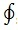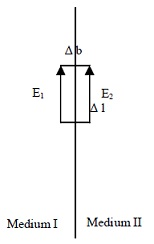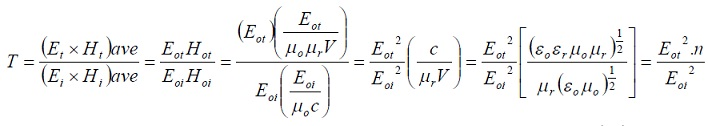#### Reflection and Refraction of electromagnetic waves, Physics tutorial

Introduction:

Reflection and refraction of electromagnetic waves:

When the plane wave is incident at boundary between two different media, part of wave is reflected and other part is refracted or transmitted. We need to find out ratio of intensity of reflected part to intensity of incident wave and ratio of intensity of refracted (transmitted) part to intensity of incident wave. Intensity is proportional to rate of energy per unit area i.e. Poynting vector. These ratios are termed as coefficients of reflection and transmission respectively. These coefficients can be determined in terms of refractive indices of media.

From equation i.e.

∇xE =-dB/dt

In integral form this equation becomesr E.dl = -d/dt∫sB.ds

Let r be rectangular loop placed along surface common to two media asIn limit that Δb→0 i.e. along interface ∫B.ds = 0, ds being surface area and product of Δl and Δb. Therefore LHS of equation equals zero and

E.dl = E1l - E2l = 0 or E1 = E2

This implies that electric field is continuous. This is boundary condition for electric field. Boundary condition for magnetic intensity can be determined from modified Ampere's law (i.e. Maxwell's 4th equation) by considering figure belowEquation can be rendered in integral form asH.dl = ∫sjf.ds + d/dt∫sD.ds

For other medium (i.e. second medium) of finite conductivity, first term in RHS of equation becomes zero. Second term is zero in limit that Δb→0 i.e. at interface as ds is product of dl and db. Therefore equation isH.dl = 0 or H1l - H2l = 0 or H1 = H2 across the interface. H is continuous across interface. From equation of Gauss law for electric field i.e.

∇.E = Ρ/ε0 or ∇.D = Ρf

Where D = ε0E - P

We can write ∇.D = 0 as there is no free charge inside cylinder placed perpendicularly to interfaceWe can write ∇.D = 0 in integration as ∫1D.ds + ∫2D.ds = 0 as total flux of electric displacement has contribution from top and bottom surfaces of cylinder as dh → 0 at interface. Flux of D is normal to surfaces 1 and 2 of cylinder. Therefore we can write

Dds1 = Dds2 implying that D is continuous

Last boundary condition is determined from Gauss law for magnetic field i.e. ∇.B = 0 which in integral form becomes ∫B.ds = 0. Using same cylinder in Figure given above, contributions of flux of magnetic field is from top and bottom surfaces of cylinder so that we can write

1B.ds + ∫2B.ds = 0

Bds1 + Bds2 = 0 where B is continuous

From the four boundary conditions i.e.

E1along = E2along

H1along = H2along

D1across = D2across, and

B1across = B2across.

Reflection and refraction (transmission) coefficients of electromagnetic waves:

Noting that in medium 1 we have only the incident and reflected waves i.e. two waves  while in medium 2 we have only refracted or transmitted wave i.e. one wave, applying the 1st boundary condition we can write:

Ei + Er = Et

The second boundary condition gives

Hi - Hr = Ht

Where subscripts i, r and t represent incident, refracted and transmitted. Note that reflected magnetic intensity, Hr, points in direction opposite direction of Hi. This can be visualized by making middle finger of left hand point in direction of propagation (z-direction) while forefinger and thumb represents E and B respectively (i.e. in x and y directions). By pointing middle finger in negative z-direction, thumb representing magnetic field is observed to be pointing downward i.e. opposite its former direction.

Using Hi = Bi0 = Ei/cμ0

As B = E/c and Hi is in free space. Substituting for

c = √1/εμ0

Hi = (ε0)1/2Ei/(μ0)1/2

Likewise Hr (also in free space) = (ε0)1/2Er/(μ0)1/2

Ht is though in medium of relative permittivity, εr and relative permeability, μr.

Therefore Ht = (ε0εr)1/2Et/(μ0μr)1/2

Equation can be written as:

((ε0)1/2Ei)/(μ01/2) - ((ε0)1/2Er)/(μ01/2) = [((ε0εr)1/2Et)/(μ0μr)1/2]

Or Ei - Er = (εr)1/2Et/(μr)1/2

μr≈1 for most dielectrics so that equation becomes

Ei - Er = (εr)1/2Et

Using amplitude of electric field of incident, reflected and transmitted waves and substituting for n = (εr)1/2 equation can be written as

Eoi - Eor = nEot

Also using amplitude of electric fields in equation we have

Eoi + Eor = Eot

By combining equations to eliminate Eot provides:

Eor/Eot = (1-n)(1+n)

Equations can also be combined to eliminate Eor. The result is

Eot/Eoi = 2/(1+n)

Ratio of reflected energy of wave to that of incident energy is referred to as reflection coefficient, R. Also ratio of transmitted energy of wave to that of incident energy is referred to as transmitted coefficient, T. Such ratios or coefficients are determined from time average of Poynting vector over one cycle for incident wave, reflected wave and transmitted wave as Poynting vector is energy per unit time per unit area. We can use combination of energy of static electric and magnetic fields to approximate energy of electromagnetic wave. Using the capacitor, energy of static electric field is achieved as follows:

Work done in transferring charge from one plate of capacitor to other plate is

dW = Vdq = (q/c)dq since V = q/c

Integrating we have

W =0q(q/c)dq = (1/2)(q2/c) or w = 1/2CV2

For parallel plate capacitor C = ε0A/d

The energy density of the electric field, UE, i.e. energy per unit volume,

UE = (ε0A/Ad.d)V2 = 1/2ε0(V/d)2 = 1/2ε0E2

(volume = Ad, E = V/d and ε0E = D) = 1/2D.E

Energy density in magnetic field is obtained as follows: let solenoid have cross sectional area. Volume Al of solenoid would store magnetic energy. Energy density is energy stored divided by Al where A is cross sectional area of solenoid and l its length. Magnetic energy dU, stored = Vidt or dU/dt = Vi or dU/dt = Vi or dU/dt = Vi or dU/dt = Li(di/dt)

As V = Ldi/dt where L is self inductance integrating,

∫dU = ∫Lidi = 1/2Li2

Energy density of magnetic field will then be, UB = (1/2)(Li2/Al)

Substituting for L = μ0n2lA and i = B/μ0n

Where n = number of turns of solenoid

UB = (1/2)(B/μ0)

Substituting for H = (B/μ0), U = (1/2)B.H and total magnetic energy is

UB = 1/2∫B.Hdv

Equations can be combined to provide energy of electromagnetic wave assuming that it does not change for varying fields. Therefore

Uem = 1/2∫(E.D + B.H)dv

For the plane wave travelling along z-axis at speed c in box of cross sectional area, A and thickness dx, sum of equations give energy density of electromagnetic wave i.e.

Uem = UE + UB = 1/2ε0E2 + 1/2(B20)

Energy Uem stored in box =(UE + UB)Adx i.e.

Substituting relation B = E/c in equation we have

So that equation is

Rate of transfer of this energy per unit area, i.e. Poynting vector, N,

N = dUem/dtA = EBdx/μ0cdt

But dx/dt = c and equation becomes N = EB/μ0 = EH

Since B/μ0 = H.

The average energy crossing unit area per second = E0H0/2 i.e. N = E0H0/2 as N is in direction of propagation and directions of E and H are orthogonal we can write,

N = E x H

By definitions of reflection coefficient, R and transmission coefficient, T given earlier,

R = (Er x Hr)ave(Ei x Hi)ave = Eor2.cμ0/Eoi2.cμ0 = Eor2/Eoi2

R = (1-n/1+n)1/2Where √εr = n and √μr ≈ 1

Using equations T = 4n/(1+n)2

Tutorsglobe: A way to secure high grade in your curriculum (Online Tutoring)